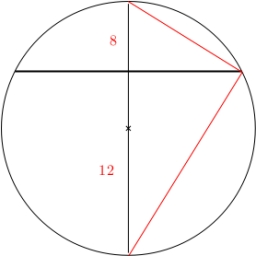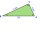# Two chords

Calculate the length of chord AB and perpendicular chord BC to circle if AB is 4 cm from the center of the circle and BC 8 cm from the center of the circle.

Correct result:

a =  16 cm
b =  8 cm

#### Solution:We would be very happy if you find an error in the example, spelling mistakes, or inaccuracies, and please send it to us. We thank you!Tips to related online calculators
Looking for help with calculating roots of a quadratic equation?
Do you have a linear equation or system of equations and looking for its solution? Or do you have quadratic equation?
Do you want to convert length units?
Pythagorean theorem is the base for the right triangle calculator.

#### You need to know the following knowledge to solve this word math problem:

We encourage you to watch this tutorial video on this math problem:

## Next similar math problems:

• Calculate 6Calculate the distance of a point A[0, 2] from a line passing through points B[9, 5] and C[1, -1].
• Parametric formCalculate the distance of point A [2,1] from the line p: X = -1 + 3 t Y = 5-4 t Line p has a parametric form of the line equation. ..
• Three pointsThree points K (-3; 2), L (-1; 4), M (3, -4) are given. Find out: (a) whether the triangle KLM is right b) calculate the length of the line to the k side c) write the coordinates of the vector LM d) write the directional form of the KM side e) write the d
• Three points 2The three points A(3, 8), B(6, 2) and C(10, 2). The point D is such that the line DA is perpendicular to AB and DC is parallel to AB. Calculate the coordinates of D.
• DodecagonCalculate the size of the smaller of the angles determined by lines A1 A4 and A2 A10 in the regular dodecagon A1A2A3. .. A12. Express the result in degrees.
• Coordinates of a centroindLet’s A = [3, 2, 0], B = [1, -2, 4] and C = [1, 1, 1] be 3 points in space. Calculate the coordinates of the centroid of △ABC (the intersection of the medians).
• Angle of the body diagonalsUsing vector dot product calculate the angle of the body diagonals of the cube.
• Determine 3Determine the distance of a point B[1, -3] from the perpendicular projection of a point A[3, -2] on a straight line 2 x + y + 1 = 0.
• Find the 10Find the value of t if 2tx+5y-6=0 and 5x-4y+8=0 are perpendicular, parallel, what angle does each of the lines make with the x-axis, find the angle between the lines?
• Vector v4Find the vector v4 perpendicular to vectors v1 = (1, 1, 1, -1), v2 = (1, 1, -1, 1) and v3 = (0, 0, 1, 1)
• Vector perpendicularFind the vector a = (2, y, z) so that a⊥ b and a ⊥ c where b = (-1, 4, 2) and c = (3, -3, -1)
• Place vectorPlace the vector AB, if A (3, -1), B (5,3) in the point C (1,3) so that AB = CO
• Ascend vs. descentWhich function is growing? a) y = 2-x b) y = 20 c) y = (x + 2). (-5) d) y = x-2
• CoordinatesDetermine the coordinates of the vertices and the content of the parallelogram, the two sides of which lie on the lines 8x + 3y + 1 = 0, 2x + y-1 = 0 and the diagonal on the line 3x + 2y + 3 = 0
• Decide 2Decide whether points A[-2, -5], B[4, 3] and C[16, -1] lie on the same line
• Vector equationLet’s v = (1, 2, 1), u = (0, -1, 3) and w = (1, 0, 7) . Solve the vector equation c1 v + c2 u + c3 w = 0 for variables c1 c2, c3 and decide weather v, u and w are linear dependent or independent
• Set of coordinatesConsider the following ordered pairs that represent a relation. {(–4, –7), (0, 6), (5, –3), (5, 2)} What can be concluded of the domain and range for this relation?